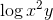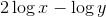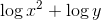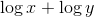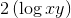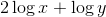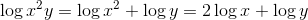# PSAT Math : How to find a ratio of exponents

## Example Questions

### Example Question #1 : How To Find A Ratio Of Exponents

Ifandare positive integers and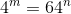, then what is the value of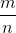?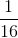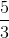Explanation:

43 = 64

Alternatively written, this is 4(4)(4) = 64 or 43 = 641.

Thus, m = 3 and n = 1.

m/n = 3/1 = 3.

### Example Question #1 : How To Find A Ratio Of Exponents

Write the following logarithm in expanded form: